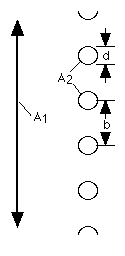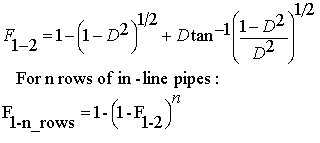Cody

# Problem 2831. Radiation Heat Transfer — View Factors (4)

View factors (aka configuration factors) are utilized in some radiation heat transfer models to estimate heat transfer rates between surfaces. In particular, the thermal energy leaving a given surface is applied to other surfaces, as appropriate, based on how much the hot surface "sees" the other surfaces. As such, view factors are purely geometrical in nature. A range of view factor formulae are available here.

For this problem, calculate the view factor from surface 1 (an infinitely long plate) to surfaces 2 (n rows of in-line pipes):The view factor for one row of pipes is F_1-2 (the first equation):The second equation is utilized for more than one row of pipes. Any of the variables can be a vector. Also, note that D = d/b, where d is the pipe diameter and b is the center-to-center spacing between pipes.

### Solution Stats

32.08% Correct | 67.92% Incorrect
Last Solution submitted on Dec 19, 2019